Скачать презентацию Combinatorial Auctions Bidding and Allocation Adapted from Noam

ae50f0c01b77e7cf743533478813be37.ppt

• Количество слайдов: 28Combinatorial Auctions (Bidding and Allocation) Adapted from Noam NisanWhat is a Combinatorial Auction? • Set of Products: • Each customer can bid: \$700 for { AND \$1200 for { \$6 for { } } OR \$8 for { } XOR \$30 for { \$3 for {ANY 3} } }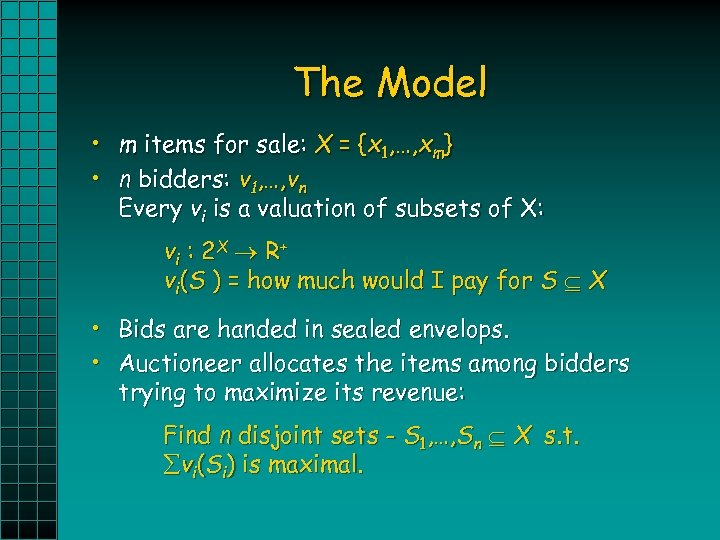The Model • m items for sale: X = {x 1, …, xm} • n bidders: v 1, …, vn Every vi is a valuation of subsets of X: vi : 2 X R + vi(S ) = how much would I pay for S X • Bids are handed in sealed envelops. • Auctioneer allocates the items among bidders trying to maximize its revenue: Find n disjoint sets - S 1, …, Sn X s. t. vi(Si) is maximal.Issues • Bidding – Expressiveness – Simplicity • Allocation – Hardness Results – Approximation Algorithms • Payment • Strategy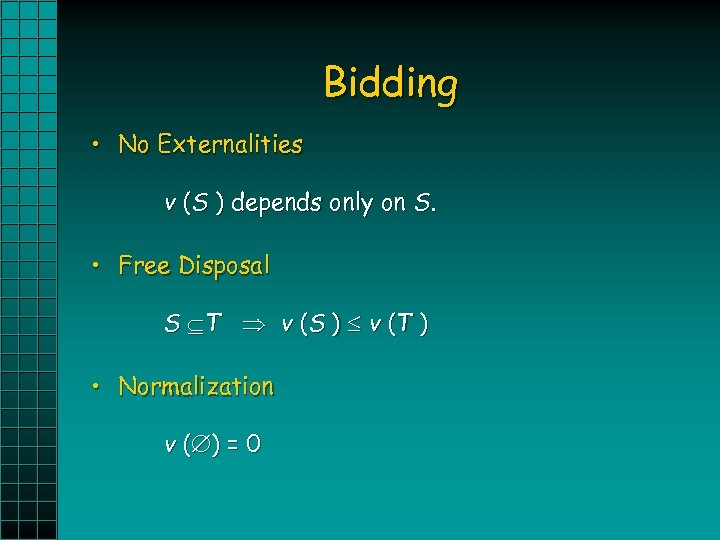Bidding • No Externalities v (S ) depends only on S. • Free Disposal S T v (S ) v (T ) • Normalization v ( ) = 0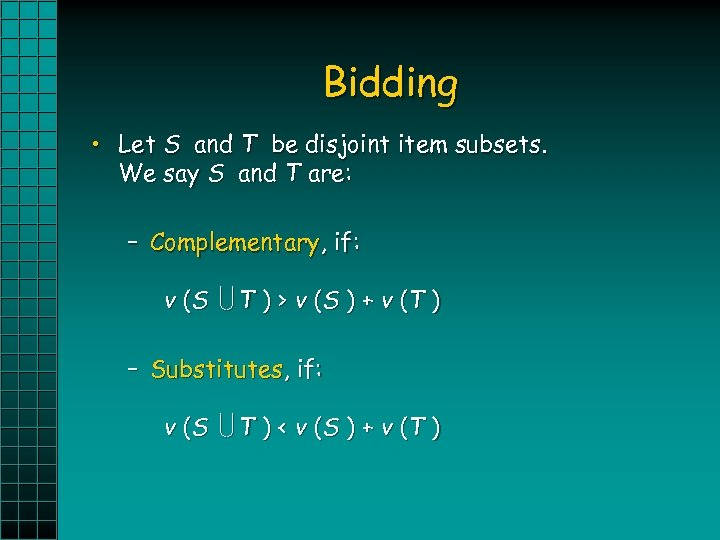Bidding • Let S and T be disjoint item subsets. We say S and T are: – Complementary, if: v (S T ) > v (S ) + v (T ) – Substitutes, if: v (S T ) < v (S ) + v (T )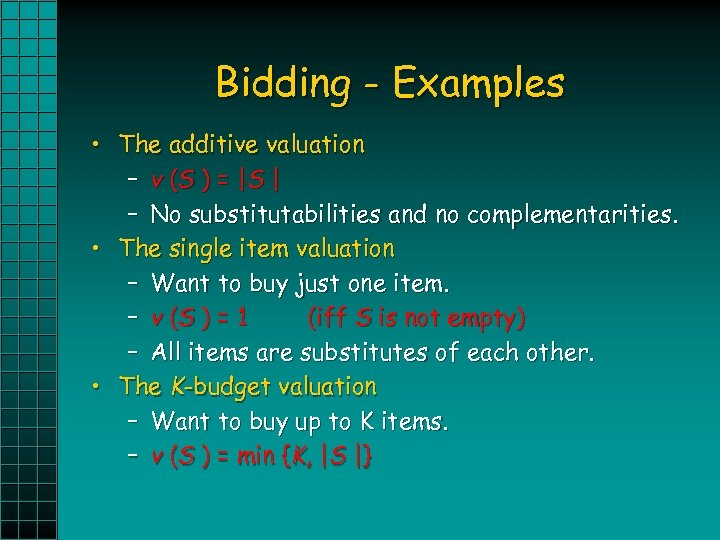Bidding - Examples • The additive valuation – v (S ) = | S | – No substitutabilities and no complementarities. • The single item valuation – Want to buy just one item. – v (S ) = 1 (iff S is not empty) – All items are substitutes of each other. • The K-budget valuation – Want to buy up to K items. – v (S ) = min {K, |S |}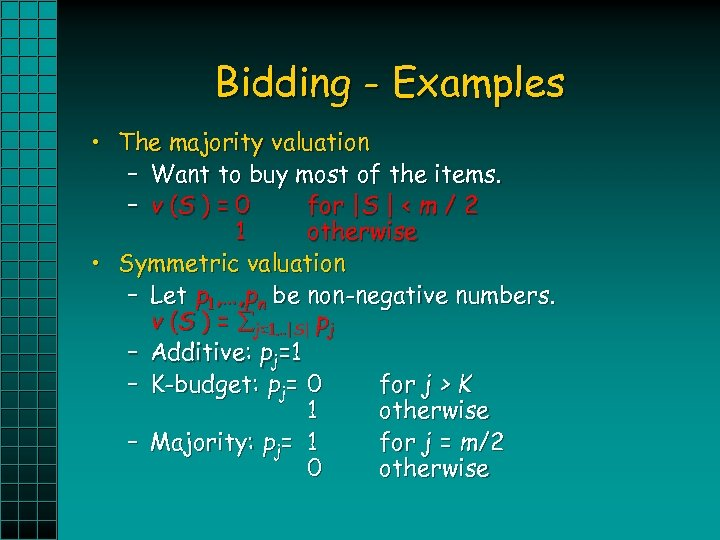Bidding - Examples • The majority valuation – Want to buy most of the items. – v (S ) = 0 for |S | < m / 2 1 otherwise • Symmetric valuation – Let p 1, …, pn be non-negative numbers. v (S ) = j=1…|S| pj – Additive: pj=1 – K-budget: pj= 0 for j > K 1 otherwise – Majority: pj= 1 for j = m/2 0 otherwise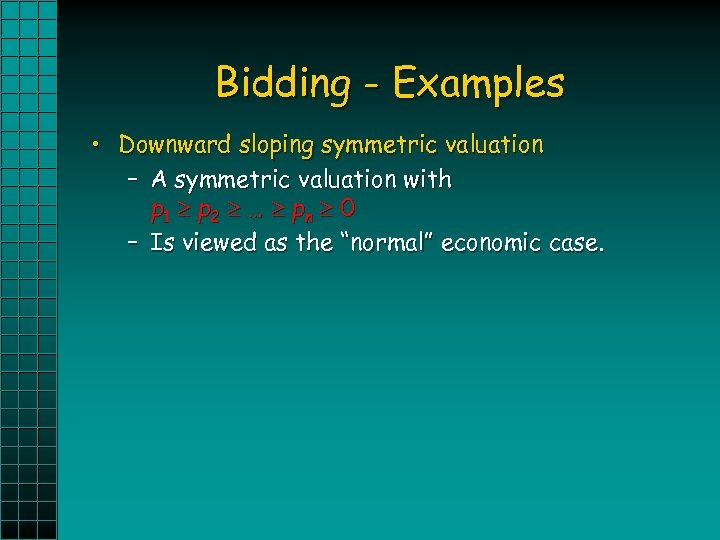Bidding - Examples • Downward sloping symmetric valuation – A symmetric valuation with p 1 p 2 … pn 0 – Is viewed as the “normal” economic case.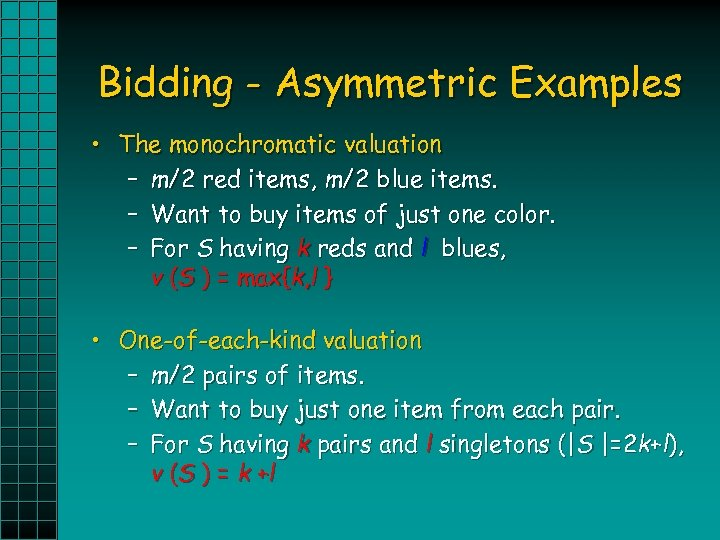Bidding - Asymmetric Examples • The monochromatic valuation – m/2 red items, m/2 blue items. – Want to buy items of just one color. – For S having k reds and l blues, v (S ) = max{k, l } • One-of-each-kind valuation – m/2 pairs of items. – Want to buy just one item from each pair. – For S having k pairs and l singletons (|S |=2 k+l), v (S ) = k + l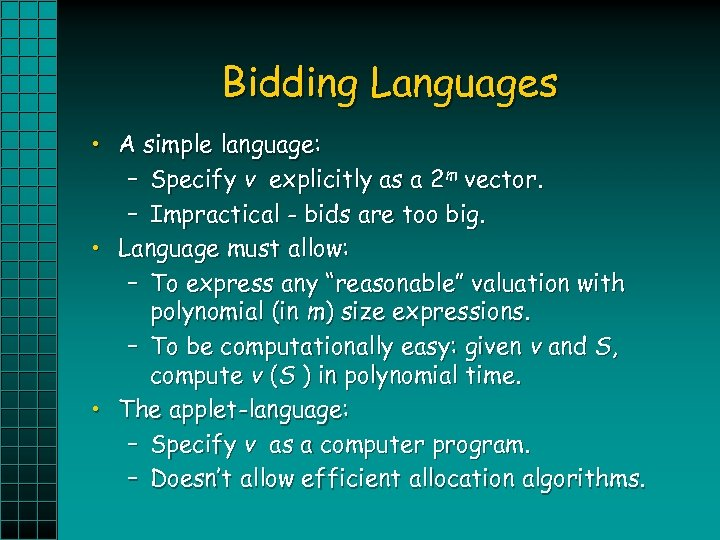Bidding Languages • A simple language: – Specify v explicitly as a 2 m vector. – Impractical - bids are too big. • Language must allow: – To express any “reasonable” valuation with polynomial (in m) size expressions. – To be computationally easy: given v and S, compute v (S ) in polynomial time. • The applet-language: – Specify v as a computer program. – Doesn’t allow efficient allocation algorithms.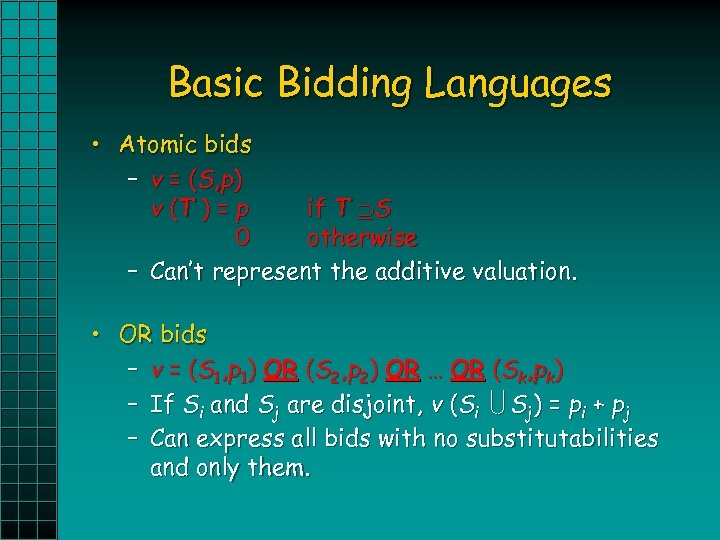Basic Bidding Languages • Atomic bids – v = ( S, p ) v (T ) = p if T S 0 otherwise – Can’t represent the additive valuation. • OR bids – v = (S 1, p 1) OR (S 2, p 2) OR … OR (Sk, pk) – If Si and Sj are disjoint, v (Si Sj) = pi + pj – Can express all bids with no substitutabilities and only them.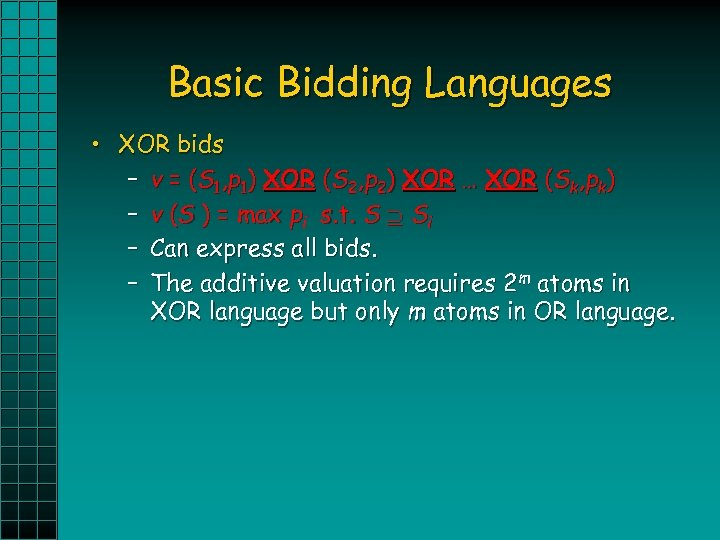Basic Bidding Languages • XOR bids – v = (S 1, p 1) XOR (S 2, p 2) XOR … XOR (Sk, pk) – v (S ) = max pi s. t. S Si – Can express all bids. – The additive valuation requires 2 m atoms in XOR language but only m atoms in OR language.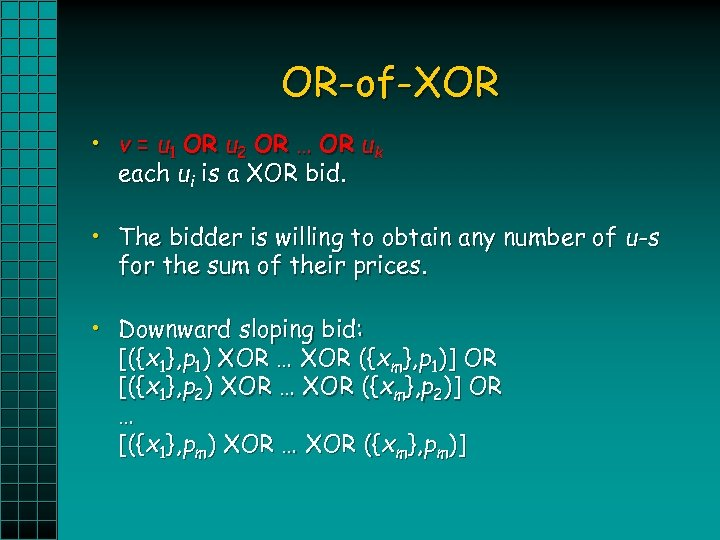OR-of-XOR • v = u 1 OR u 2 OR … OR uk each ui is a XOR bid. • The bidder is willing to obtain any number of u-s for the sum of their prices. • Downward sloping bid: [({x 1}, p 1) XOR … XOR ({xm}, p 1)] OR [({x 1}, p 2) XOR … XOR ({xm}, p 2)] OR … [({x 1}, pm) XOR … XOR ({xm}, pm)]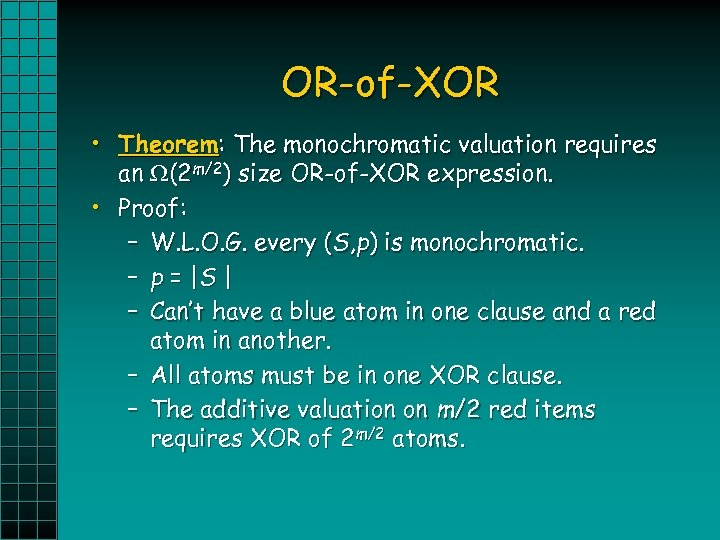OR-of-XOR • Theorem: The monochromatic valuation requires an (2 m/2) size OR-of-XOR expression. • Proof: – W. L. O. G. every (S, p) is monochromatic. – p = |S | – Can’t have a blue atom in one clause and a red atom in another. – All atoms must be in one XOR clause. – The additive valuation on m/2 red items requires XOR of 2 m/2 atoms.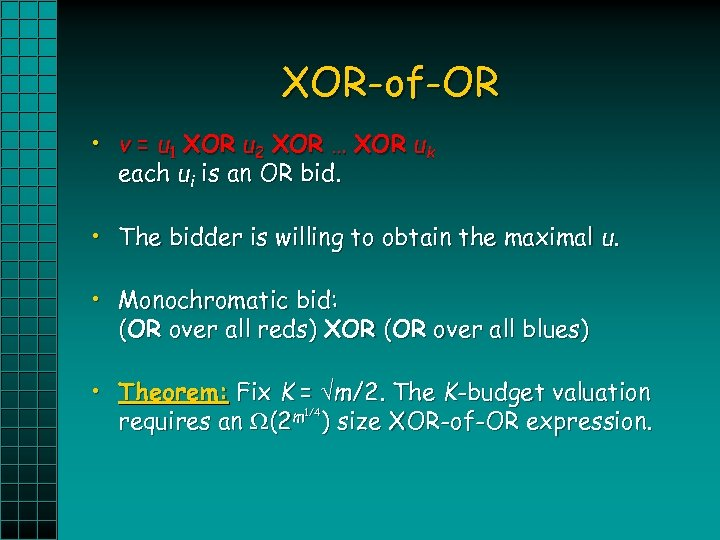XOR-of-OR • v = u 1 XOR u 2 XOR … XOR uk each ui is an OR bid. • The bidder is willing to obtain the maximal u. • Monochromatic bid: (OR over all reds) XOR (OR over all blues) • Theorem: Fix K = m/2. The K-budget valuation 1/4 requires an (2 m ) size XOR-of-OR expression.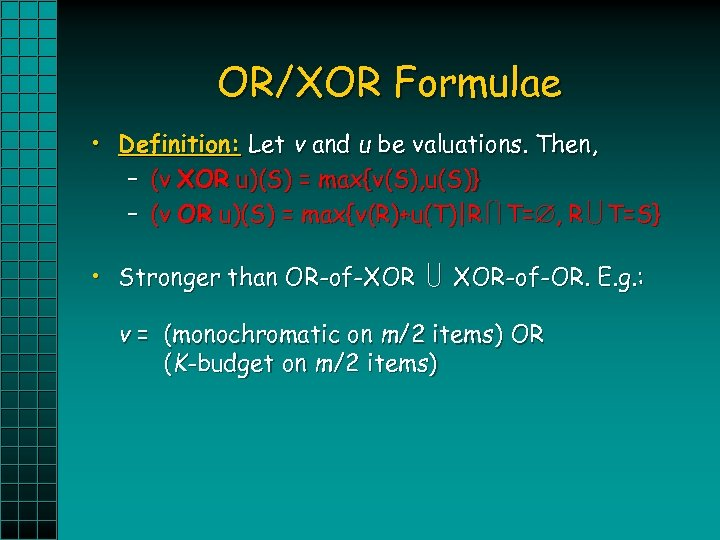OR/XOR Formulae • Definition: Let v and u be valuations. Then, – (v XOR u)(S) = max{v(S), u(S)} – (v OR u)(S) = max{v(R)+u(T)|R T= , R T=S} • Stronger than OR-of-XOR XOR-of-OR. E. g. : v = (monochromatic on m/2 items) OR (K-budget on m/2 items)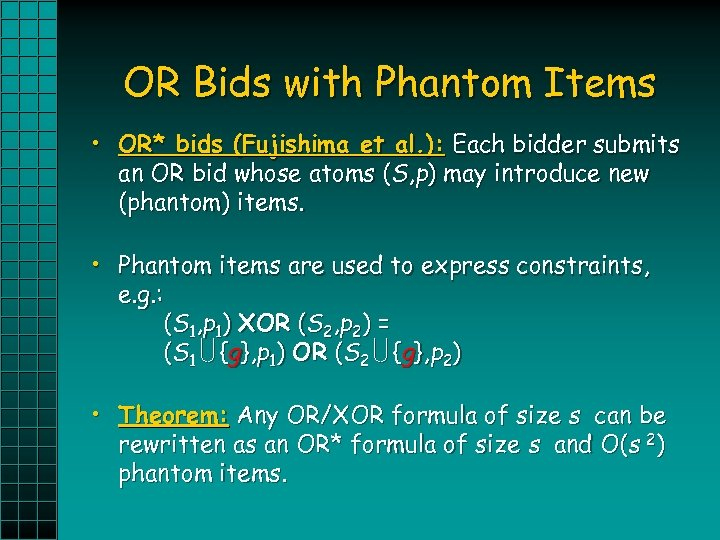OR Bids with Phantom Items • OR* bids (Fujishima et al. ): Each bidder submits an OR bid whose atoms (S, p) may introduce new (phantom) items. • Phantom items are used to express constraints, e. g. : (S 1, p 1) XOR (S 2, p 2) = (S 1 {g}, p 1) OR (S 2 {g}, p 2) • Theorem: Any OR/XOR formula of size s can be rewritten as an OR* formula of size s and O(s 2) phantom items.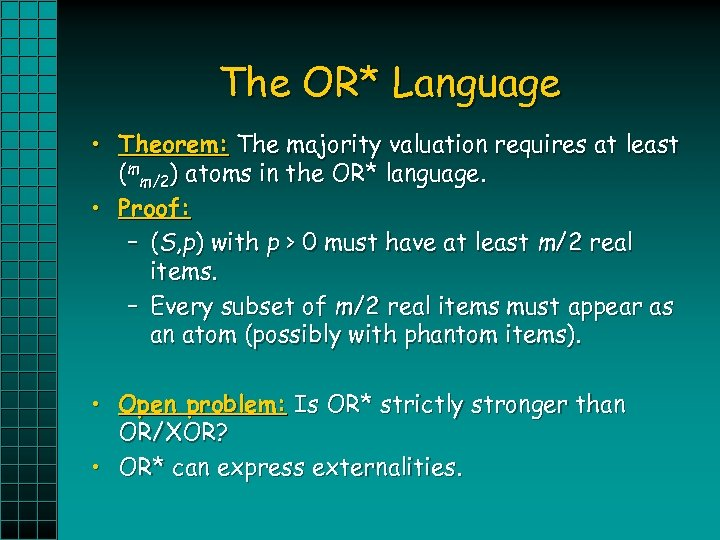The OR* Language • Theorem: The majority valuation requires at least (mm/2) atoms in the OR* language. • Proof: – (S, p) with p > 0 must have at least m/2 real items. – Every subset of m/2 real items must appear as an atom (possibly with phantom items). • Open problem: Is OR* strictly stronger than OR/XOR? • OR* can express externalities.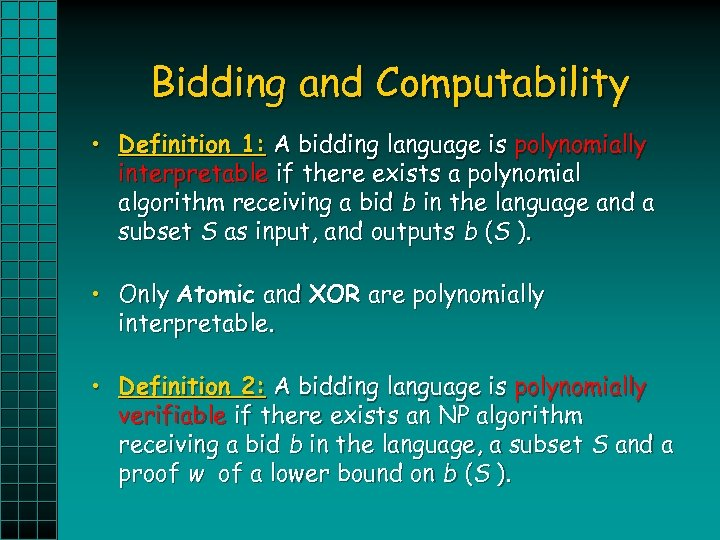Bidding and Computability • Definition 1: A bidding language is polynomially interpretable if there exists a polynomial algorithm receiving a bid b in the language and a subset S as input, and outputs b (S ). • Only Atomic and XOR are polynomially interpretable. • Definition 2: A bidding language is polynomially verifiable if there exists an NP algorithm receiving a bid b in the language, a subset S and a proof w of a lower bound on b (S ).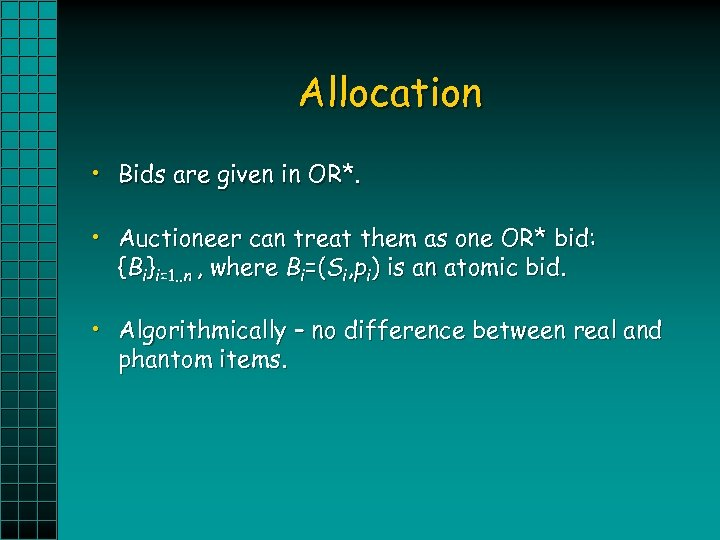Allocation • Bids are given in OR*. • Auctioneer can treat them as one OR* bid: {Bi}i=1. . n , where Bi=(Si, pi) is an atomic bid. • Algorithmically – no difference between real and phantom items.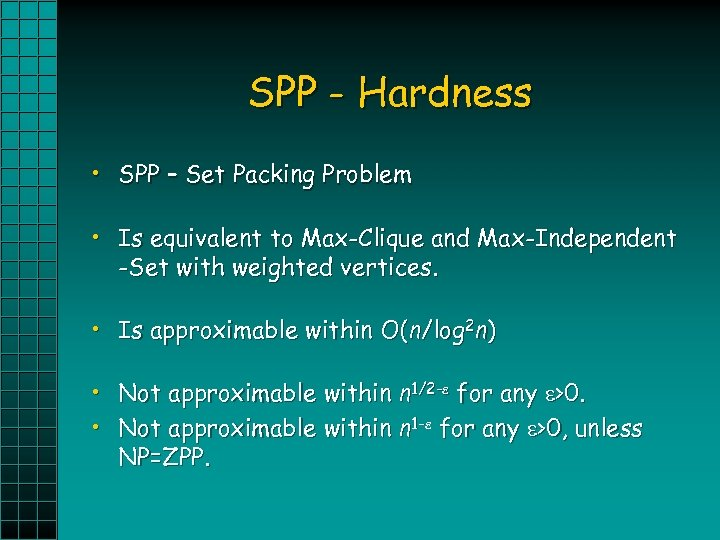SPP - Hardness • SPP – Set Packing Problem • Is equivalent to Max-Clique and Max-Independent -Set with weighted vertices. • Is approximable within O(n/log 2 n) • Not approximable within n 1/2 - for any >0. • Not approximable within n 1 - for any >0, unless NP=ZPP.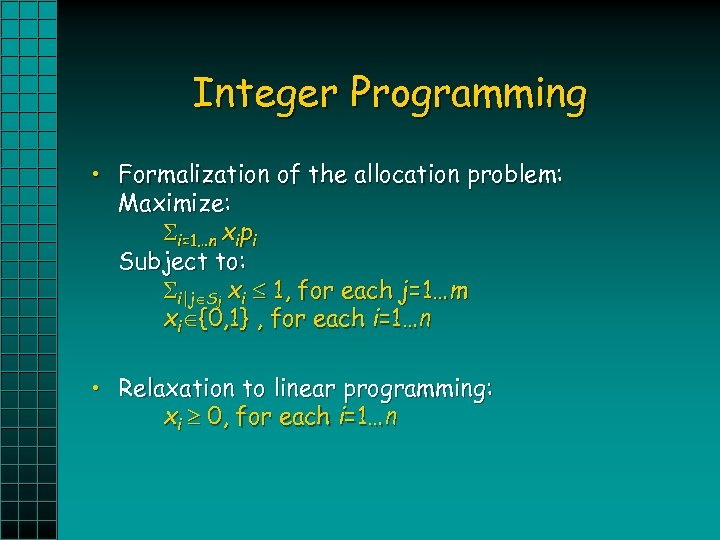Integer Programming • Formalization of the allocation problem: Maximize: i=1…n xipi Subject to: i|j Sj xi 1, for each j=1…m xi {0, 1} , for each i=1…n • Relaxation to linear programming: xi 0, for each i=1…n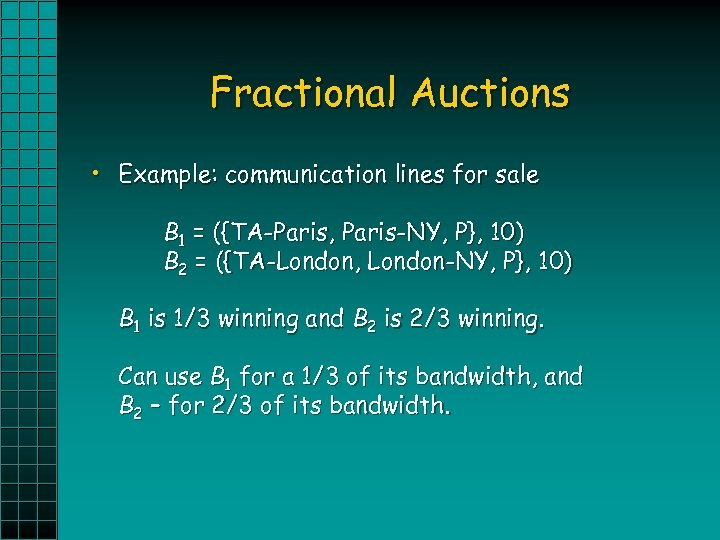Fractional Auctions • Example: communication lines for sale B 1 = ({TA-Paris, Paris-NY, P}, 10) B 2 = ({TA-London, London-NY, P}, 10) B 1 is 1/3 winning and B 2 is 2/3 winning. Can use B 1 for a 1/3 of its bandwidth, and B 2 – for 2/3 of its bandwidth.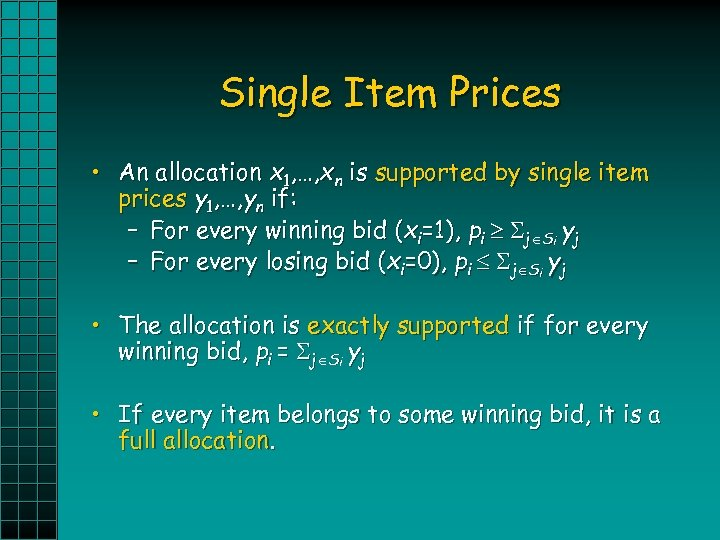Single Item Prices • An allocation x 1, …, xn is supported by single item prices y 1, …, yn if: – For every winning bid (xi=1), pi j Si yj – For every losing bid (xi=0), pi j Si yj • The allocation is exactly supported if for every winning bid, pi = j Si yj • If every item belongs to some winning bid, it is a full allocation.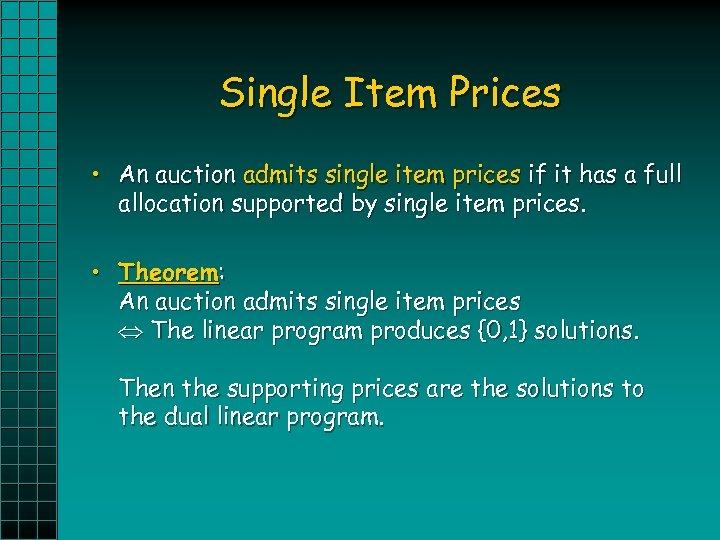Single Item Prices • An auction admits single item prices if it has a full allocation supported by single item prices. • Theorem: An auction admits single item prices The linear program produces {0, 1} solutions. Then the supporting prices are the solutions to the dual linear program.Single Item Prices - Example • Bidder #1: ({A, P}, 5)XOR({B, P}, 6) ({A}, 5) OR ({B}, 6) • Bidder #2: ({B}, 3) • Bidder #1 wins A for 5\$. Bidder #2 wins B for 3\$. • Supporting prices: A = 2\$, B = 3\$, P = 3\$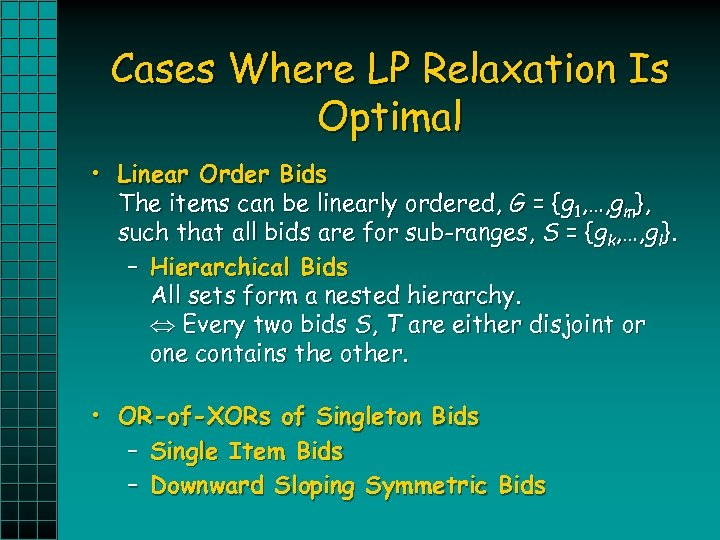Cases Where LP Relaxation Is Optimal • Linear Order Bids The items can be linearly ordered, G = {g 1, …, gm}, such that all bids are for sub-ranges, S = {gk, …, gl}. – Hierarchical Bids All sets form a nested hierarchy. Every two bids S, T are either disjoint or one contains the other. • OR-of-XORs of Singleton Bids – Single Item Bids – Downward Sloping Symmetric Bids